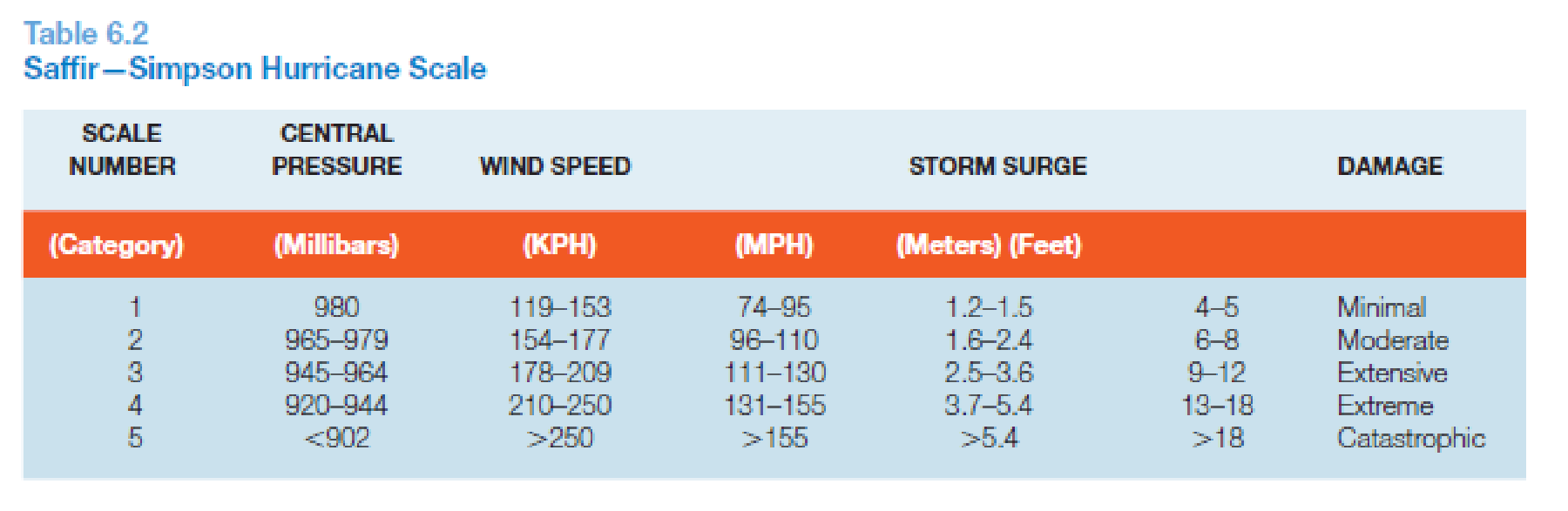Chapter 6, Problem 3PA

Chapter
Section
Textbook Problem

Wind speeds are sometimes given in knots (nautical miles per hour) instead of statute miles per hour. A nautical mile (used mainly by sailors and pilots) equals 6,080 feet, slightly longer than a statute mile at 5,280 feet. Being longer than a statute mile, a knot is a little faster than one mile per hour. The conversion from miles per hour to knots is:Knots = mph × 1.151Using Table 6.2, convert the ranges of wind speeds for hurricane categories 1 through 5 from miles per hour to knots.To determine

The values of wind speeds in knots for hurricane categories 1 to 5 given in miles per hour.

Explanation

It is given in the question that 1knot=1.151mph.

Therefore:

1mph=11.151knots=0.8689knots

Category 1

Speed in mph= 74 to 95

Speed in knots=(74×0.8689)knots to (95×0.8689)knots=64 to 83knots ­­

Category 2

Speed in mph= 96 to 110

Speed in knots=(96×0.8689)knots to (110×0.8689)knots=83.4 to 96knots

For approximation, the 83

Still sussing out bartleby?

Check out a sample textbook solution.

See a sample solution

The Solution to Your Study Problems

Bartleby provides explanations to thousands of textbook problems written by our experts, many with advanced degrees!

Get Started

Find more solutions based on key concepts